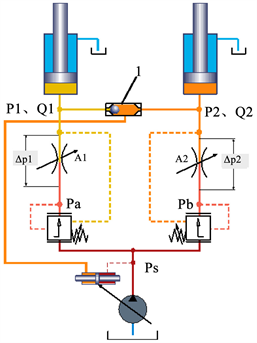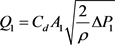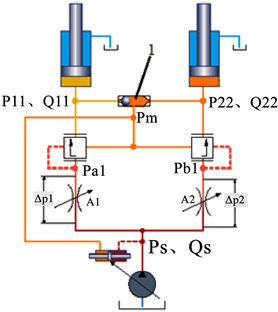# 负载敏感技术在工程机械上的应用Application of Load Sensitive Technology in Engineering Machinery

• 全文下载: PDF(759KB)    PP.109-113   DOI: 10.12677/IJFD.2018.64014
• 下载量: 390  浏览量: 936

This thesis takes load-sensing technology as the research object. The thesis analyzes the difference between pre-valve load sensitivity technology and post-valve load sensitivity technology systematic, which provides a theoretical basis for engineering machinery to select load-sensitive technology.

1. 前言

2. 阀前补偿的负载敏感系统Figure 1. Schematic diagram of pre-valve compensation load sensing system(1)

${Q}_{2}={C}_{d}{A}_{2}\sqrt{\frac{2}{\rho }\Delta {P}_{2}}$ (2)

$\Delta {P}_{1}={P}_{a}-{P}_{1}$ (3)

$\Delta {P}_{2}={P}_{b}-{P}_{2}$ (4)

${P}_{a}={P}_{1}+\Delta {X}_{1}$ (5)

${P}_{b}={P}_{2}+\Delta {X}_{2}$ (6)

$\Delta {P}_{1}=\Delta {X}_{1}$ (7)

$\Delta {P}_{2}=\Delta {X}_{2}$ (8)

2.1. 流量非饱和状态

2.2. 流量饱和状态

${P}_{a}-{P}_{1}>\Delta {X}_{1}$ ，此工作联压力补偿阀处于有效状态，此联的流量仍然由主阀芯节流面积 ${A}_{1}$ 、和压力补偿阀弹簧所提供的 $\Delta {X}_{1}$ 决定；

${P}_{a}-{P}_{1}<\Delta {X}_{1}$ ，此工作联压力补偿阀处于失效状态，此时作用于压力补偿阀两端的压差已不足以克服压力补偿阀弹簧腔的弹簧力，导致压力补偿阀处于完全开启状态，此状态下的工作联相当于两个节流阀相串联(压力补偿阀节流和主阀芯节流)，其工作联压差 $\Delta P={P}_{s}-{P}_{1}$ ；此状态下 ${P}_{1}$ 越大， $\Delta P$ 越小，节流面积是压力补偿阀节流和主阀芯节流面积的串联，其有效面积是一定值，这样就导致负载压力 ${P}_{1}$ 越大，流量越小。

${P}_{a}-{P}_{1}=\Delta {X}_{1}$ ，此状态下，工作联处于临界状态，因外负载的不稳定性，此状态下的压差关系处于不稳定状态，可导致流量的不稳定，进而影响系统的稳定性，在工程机械液压系统中要避免这种现象。

3. 阀后补偿的负载敏感系统Figure 2. Schematic diagram of the post-valve compensation load sensing system

${Q}_{11}={C}_{d}{A}_{1}\sqrt{\frac{2}{\rho }\Delta {P}_{1}}$ (9)

${Q}_{22}={C}_{d}{A}_{2}\sqrt{\frac{2}{\rho }\Delta {P}_{2}}$ (10)

$\Delta {P}_{1}={P}_{s}-{P}_{a1}$ (11)

$\Delta {P}_{2}={P}_{s}-{P}_{b1}$ (12)

${P}_{a1}={P}_{m}+{P}_{k}$ (13)

${P}_{b1}={P}_{m}+{P}_{k}$ (14)

${P}_{k}$ ——压力补偿阀弹簧腔由弹簧所产生的压力(阀后补偿多路阀中压力补偿阀弹簧一样)。

3.1. 流量非饱和状态

3.2. 流量饱和状态

4. 结论

1) 在流量非饱和状态下，无论单动作或复合动作，阀前负载敏感与阀后负载敏感技术均能满足其性能要求，从价格、可靠性、节能等方面考虑，建议采用阀前负载敏感技术。

2) 在流量饱和状态下，虽然有复合动作的需求，但对复合动作的协调性方面没有特殊要求或无要求，阀前负载敏感和阀后均能满足系统要求，建议采用阀前负载敏感技术。

3) 在流量饱和状态下，对复合动作的协调性有严格要求，在此工况下阀前敏感技术失效，只能采用阀后敏感技术。

  张立杰, 王力航, 李德新, 王帅. 负载敏感阀前补偿系统原理分析[J]. 液压与气动, 2015(7): 63.  马春峰, 隋冬枝. 阀前补偿和阀后补偿的特点及应用[J]. 煤矿机械, 2014, 35(8): 208.  张海平. 液压速度控制技术[M]. 北京: 机械工业出版社, 2014.  景俊华. 负载敏感系统的原理及其应用[J]. 流体传动与控制, 2010(6): 21-24.  王中伟. 液压负载敏感系统分析及其研究[J]. 科技创新与应用, 2014(8): 7-8.